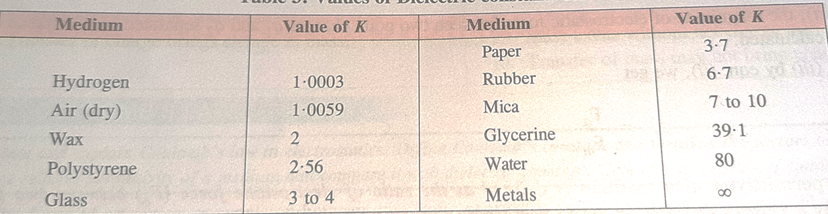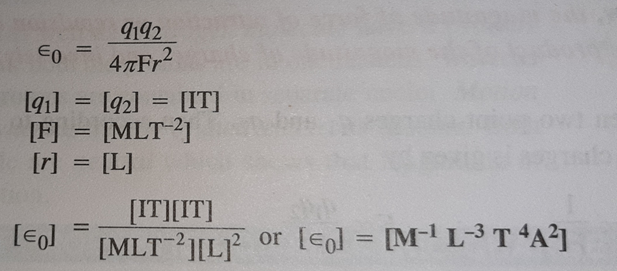For online classes & notes, Contact: quantaphysics3@gmail.com
Join Telegram: quantaphysics.com

COULOMB’S LAW:

It states that the electro-static force of attraction or repulsion between two charged bodies is directly proportional to the product of their charges and varies inversely as the square of the distance between the two bodies.

F = Kq1q2/r2

Here, K=Coulomb’s Constant

K = 1/4πε0 = 9×109 Nm2C -2 (in free space)

Fa =1/4πε0 × q1q2/r2

ε0=Permittivity of free space=8.854 × 10 -12 C2 N-1 m-2

(Permittivity is a measure of how easy or difficult it is to form an electric field inside of a medium.)

Coulomb’s law for a medium other than air can be written as,

Fm=1/4πε × q1q2/r2

ε= Permittivity of medium.

Relative Permittivity (εr) or Dielectric Constant (K):

Ø  The relative Permittivity of a medium is defined as the ratio of electrostatic force (Fa) between two point charges separated by a certain distance in air or vacuum to the electrostatic force (Fm) between the same two point charges separated by the same distance in a medium.

εr = Fa/ Fm

Ø  The relative permittivity (εr) of a medium is defined as the ratio between its permittivity of the medium (ε) and the permittivity (ε0) of the free space.

εr = ε/ε0

Ø  Dielectric Constant of a medium decreases with increase in temperature. Value of K for water at 200C=80 & K for water at 250C=78.5Value of Dielectric Constant

BDIMENSIONAL FORMULA

Characteristics of Coulomb’s Force:

• ü  It is a central force that is it acts along a line joining the centres of the particles or bodies.
• ü  The force is spherically symmetric as it is a function of r (distance between charges) only.
• ü  It obeys inverse square law (F α 1/r2).
• ü  The force may be attractive or repulsive.
• ü  The force is not affected by the presence of other charges. Hence electrostatic force is a two-body interaction.
• For online classes & notes, Contact: quantaphysics3@gmail.com
Join Telegram: quantaphysics.com

Contact for all Doubts & Online Classes: quantaphysics3@gmail.com